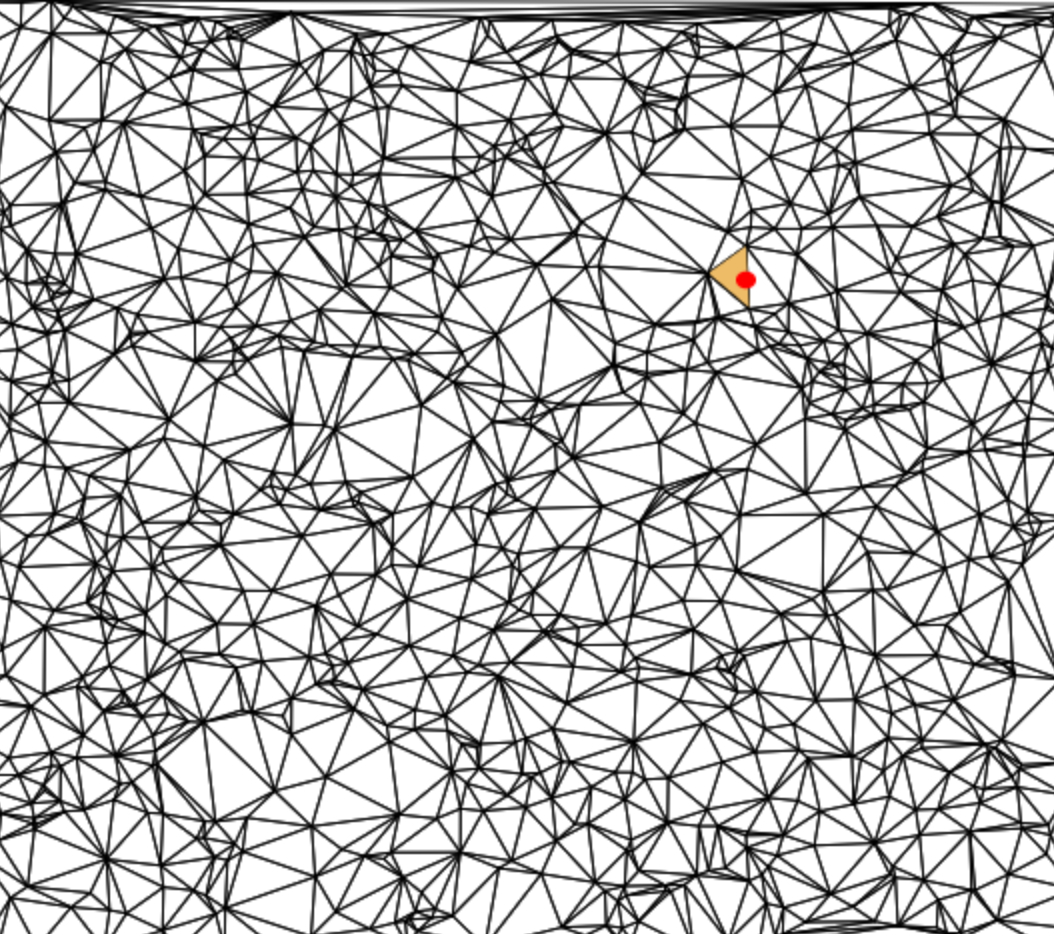# point-in-region

Quickly and robustly determines which region contains a given query point

## Stats

StarsIssuesVersionUpdatedCreatedSize
point-in-region
2521.0.09 years ago9 years agopoint-in-region
Locates a point in a collection of regions. Point location is exact, takes O(log(n)) time, and the data structure has a space requirement of O(n log(n)).
Example
``````//First create a list of vertices
var vertices = [
[0, 0],
[1, 0],
[1, 1],
[0, 1],
[2, 0],
[3, 0],
[3, 1],
[2, 1],
[2.25, 0.25],
[2.75, 0.25],
[2.75, 0.75],
[2.25, 0.75]
]

//Regions are defined by lists of loops of vertex indices
var regions = [
//First region, just a square, one loop
[
[0, 1, 2, 3]
],
//Second region, square with a hole in the middle
[
[4, 5, 6, 7],
[11, 10, 9, 8]    //Note inner loop has opposite orientation
]
]

//Now we create the data structure
var classifyPoint = require("point-in-region")(vertices, regions)

//And we can use it to classify which region contains a given point
var assert = require("assert")
assert.equal(classifyPoint([0.5, 0.5]), 0)
assert.equal(classifyPoint([2.1, 0.1]), 1)
assert.equal(classifyPoint([100000, 10000]), -1)  //Outside points return -1
assert.equal(classifyPoint([2.5, 0.5]), -1)  //Point in center hole is outside region``````

Here is an in browser demo you can try out yourself:Install
``npm install point-in-region``
API

### `var classify = require("point-in-region")(positions, regions)`

Preprocesses a collection of regions to answer point location queries efficiently.
• `positions` is a list of vertex positions for each of the regions
• `regions` is a list of regions encoded as lists of clockwise oriented loops of indices

Returns A point membership classification function for the region set
Note The regions must obey certain topological and geometric properties for this classification to be correct. Specifically:
• Any two edges are either disjoint, identical, or meet at exactly one common end point
• The interior of each edge touches at most two regions

### `classify(point)`

Returns the index of the region containing `point`
• `point` is a 2D point encoded as a length 2 array

Returns The index of the region containing `point` or `-1` if it is not in any region.
Credits
(c) 2014 Mikola Lysenko. MIT License